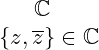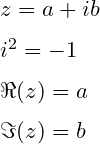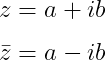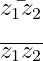# How to write complex number(ℂ) and imaginary part in LaTeX?

Mathematically, the set of complex number is denoted by the Blackboard bold ℂ.

And the best way to represent this Blackboard bold symbol in latex is to use the mathbb command.

 Symbol Complex number Type Set Package asmmath, amssymb Command \mathbb{C} Example \mathbb{C} → ℂ
\documentclass{article}
\begin{document}
$\mathbb{C}$
$\{z,\overline{z}\} \in \mathbb{C}$
\end{document}

Output :Equations of a complex number have two parts, real and imaginary. The real part is represented by the symbol and the imaginary part by the symbol.

\documentclass{article}
\begin{document}
$z=a+ib$
$i^2=-1$
$\Re(z)=a$
$\Im(z)=b$
\end{document}

Output :## Real and imaginary part in LaTeX

The real and complex part has been denoted in different ways in different books. So, there are some morphological differences. You look at this table below

Package Commands
Default \Re, \Im
amsmath \operatorname
amsfonts \mathfrak
physics \Re, \Im

Although both \operatorname{arg1}{arg2} and \mathfrak{arg1}{arg2} commands have the same syntax, they are completely different in structure compared to other commands.

In this case you need to pass two arguments like arg1, arg2. Where the first is abbreviation of real part or imaginary part and second is value.

\documentclass{article}
\usepackage{amsmath,amsfonts}
\begin{document}
$\Re(z),\Im(z)$
$\operatorname{Re}(z), \operatorname{Im}(z)$
$\mathfrak{Re}(z), \mathfrak{Im}(z)$
\end{document}

Output :And the same command is present in the physics package to define complex and real parts. For example

\documentclass{article}
\usepackage{physics}
\begin{document}
$z=a+ib$
$\Re{z}=a$
$\Im{z}=b$
\end{document}

Output :## Complex conjugation in LaTeX

If you denote the complex number by z, then the conjugation of the complex number has to be denoted by z bar.

\documentclass{article}
\begin{document}
$z=a+ib$
$\bar{z}=a-ib$
\end{document}

Output :But, you should not use the \bar command to use this bar symbol on more than one character. Because the bar symbol will not cover more than one character.

In this case, you need to use the \overline command. As a result, the bar symbol will be positioned responsibly on more than one character.

\documentclass{article}
\usepackage{physics}
\begin{document}
$\bar{z_{1}z_{2}}$
$\overline{z_{1}z_{2}}$
\end{document}

Output :#### Md Jidan Mondal

LaTeX expert with over 10 years of experience in document preparation and typesetting. Specializes in creating professional documents, reports, and presentations using LaTeX.